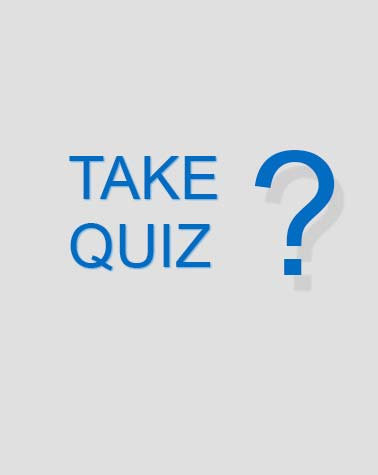Gebhardt---atoms And Atomic Structure Notes Check

5 Questions | Total Attempts: 153Settings• 1.
What are most atoms made of?
• A.

Molecules

• B.

Water

• C.

Protons, neutrons and electrons

• D.

None of the above

• 2.
What is the charge given to electrons?
• A.

Negative

• B.

No charge

• C.

Positive

• D.

None of the above

• 3.
Which of the following has the smallest mass?
• A.

Proton

• B.

Electron

• C.

Neutron

• D.

They all have the same mass and there is no difference

• 4.
Sodium is an element that makes up table salt.  Its atomic number is 11 and its atomic mass is 22.999.  How many neutrons does this Sodium atom have?
• A.

10

• B.

11

• C.

12

• D.

13

• 5.
Using the sodium: Atomic number of 11 and Atomic Mass of 22.999; how many electrons would Sodium have?
• A.

10

• B.

11

• C.

12

• D.

13Back to top